# Square (Geometry)

A Square is a flat shape with 4 equal sides and every angle is a right angle (90°)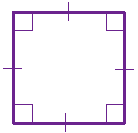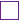means "right angle"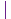show equal sides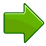All sides are equal in lengthEach internal angle is 90°Opposite sides are parallel (so it is a Parallelogram).

Play with a square:

A square also fits the definition of a rectangle (all angles are 90°), a rhombus (all sides are equal length), a parallelogram (opposite sides parallel and equal in length) and a regular polygon(all angles equal and all sides equal). What a hero!

## Area of a Square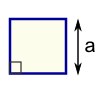The Area is the side length squared: Area = a2 = a × a

### Example: A square has a side length of 6 m, what is its Area?

Area = 6 m × 6 m = 36 m2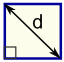The Area is also half of the diagonal squared: Area = d22

## Perimeter of a Square

The Perimeter is the distance around the edge.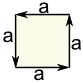The Perimeter is 4 times the side length: Perimeter = 4a

### Example: A square has a side length of 12 cm, what is its Perimeter?

Perimeter = 4 × 12 cm = 48 cm

## Diagonals of a Square

A square has two diagonals, they are equal in length and intersect in the middle.The Diagonal is the side length times the square root of 2: Diagonal "d" = a × √2

### Example: A square has a side length of 5 m, what is the length of a diagonal?Diagonal Length = a × √2
= 5 × 1.41421...
= 7.071 m (to 3 decimals)

## Calculator

Enter the side length, area, diagonal or perimeter and the other values are calculated live.